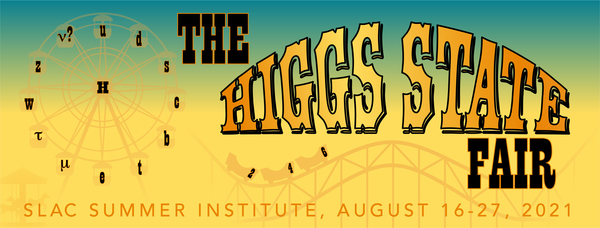#49th SLAC Summer Institute (SSI 2021)

16-27 August 2021
SLAC
America/Los_Angeles timezone

## Optimization of sensitivity for the Di-Higgs search in $b\bar{b}l\nu qq$ final state in the LHC data recorded by the ATLAS detector in $pp$ collisions at $\sqrt{s}$ = 13 TeV

Not scheduled
20m
ZOOM videoconf (SLAC)

### Speaker

Pratik Kafle (Reed College)

### Description

We present a study to optimize the search for the Di-Higgs decay produced from gluon-gluon fusion with $b\bar{b}qql\nu$ as the final state in the ATLAS experiment at the LHC using Monte Carlo simulated sample. A big challenge in observing this event is due to enormous background contamination, the dominant of which is due to the decay of $t\bar{t}$ decay. We use ROOT and its built-in multivariate analysis toolkit (TMVA) to run a sensitivity estimate using the Boosted Decision Tree algorithm to discriminate signal from the $t\bar{t}$ background. The performance of the algorithm to discriminate the background from the signal is based on the Signal significance, which is defined as $S/\sqrt{S+B}$.

### Primary author

Pratik Kafle (Reed College)